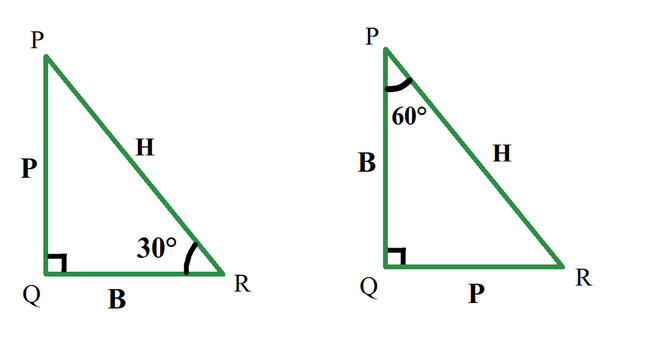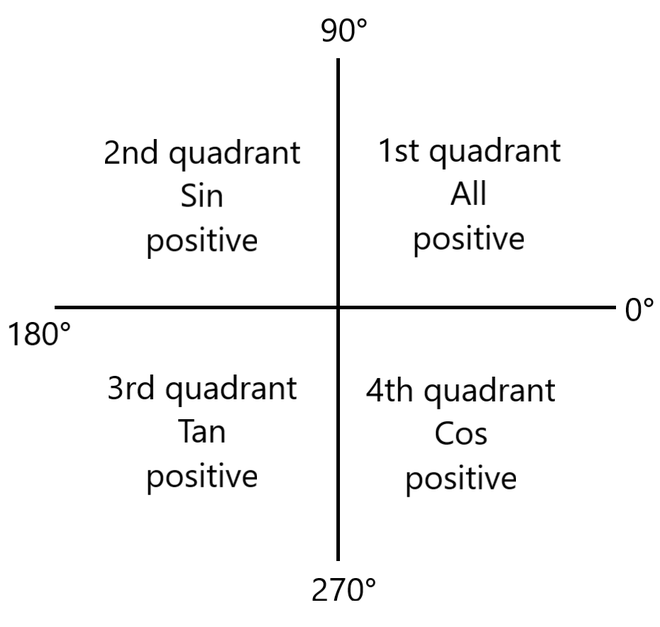GeeksforGeeks App
Open AppBrowser
Continue

## Related Articles

Trigonometry is an important branch of mathematics, it deals with the relationship of sides with angles in a right-angle triangle. Trigonometry is important in physics also, it is used to find, the height of towers, the distance between stars, or in the Navigation systems. Trigonometry is predicated on the principle that “If two triangles have an equivalent set of angles then their sides are within the same ratio”. Side lengths are often different but side ratios are equivalent.

### Trigonometric Functions

Trigonometric functions or circular functions or trigonometric ratios show The relationship of angles and sides. These trigonometric ratios are obtained by taking ratios of sides. We have six trigonometric ratios Sin, Cos, Tan, Cosec, Sec, Cot.

• sin A = Perpendicular / Hypotenuse
• cos A = Base / Hypotenuse
• tan A = Perpendicular / Base
• cot A = Base / Perpendicular
• sec A = Hypotenuse / Base
• cosec A = Hypotenuse / Perpendicular.

Here, A is that the angle opposite to the perpendicular side. Let’s see what the Perpendicular, base, and hypotenuse of a right triangle are,

1. Perpendicular: The side ahead of the angle is perpendicular. In this case, the side in front of 30 deg is called it’s perpendicular.
2. Base: A base is one among the edges which contain an angle, except hypotenuse.
3. Hypotenuse: It is a side opposite to 90°. It is the largest side.

Note: Perpendicular and base changes as angle changes. During a triangle, a side is perpendicular for an angle, but an equivalent side may be a base for an additional angle, but the hypotenuse remains an equivalent because it’s a side opposite to angle 90°.As shown within the above diagram for an equivalent triangle if considered angle 30° the perpendicular is that the side PQ, but if considered angle 60° the perpendicular is the side QR.

### What is all sin tan cos rule?

All sin tan cos rule tells about the sign(+ve or -ve) of trigonometric ratios. When trigonometric ratios are calculated of all angles between 0° to 360° or 0° to 2π we also have to take care of the sign(+ve or -ve) of Sine, Cos, and tan. Since the other 3 trigonometric ratios are reciprocal of these three their sign is decided by these three ratios.

All sin tan cos rule says that,

1. When angle θ lies in the first quadrant 0° ≤ θ ≤ 90° all three ratios i.e. Sine, cos, and tan are positive with their reciprocals.
2. When angle θ lies in the second quadrant i.e. 90° ≤ θ ≤ 180° sin and its reciprocal cosec are positive and other ratios tan and cos are negative in the second quadrant.
3. When angle θ lies in the third quadrant i.e. 180° ≤ θ ≤ 270° tan and its reciprocal cot are positive and other ratios sin and cos are negative in the third quadrant.
4. When angle θ lies in the fourth quadrant i.e. 270° ≤ θ ≤ 360° cos and its reciprocal sec are positive and other ratios sin and tan are negative in the fourth quadrant.### Sample Problems

Question1. Find the sign of Sin(150°), Sin(255°), Cos(160°), Cos(320°), tan(170°), tan (350°).

Solution:

According to all sin tan cos rule,

Sin(150°) = +ve

Sin(225°) = -ve

Cos(160°) = -ve

Cos(320°) = +ve

tan(170°) = +ve

tan(350°) = -ve

Question2: find the value of sin(225°), tan(225°), cos(225°).

Solution:

225° lies in the 3rd quadrant, According to all sin tan cos rule in 3rd quadrant tan is positive and sin and cos are negative.

sin(225°) = sin(180° + 45°)

Sin(225°) = -1/√2

tan(225°) = sin(180° + 45°)

Tan(225°) = 1

Cos(225°) = Cos(180° + 45°)

cos(225°) = -1/√2

Solution 3: Find Value of Sin(225°) – Cos(315°).

Solution:

Sin(225°) = -1/√2

Cos(315°) = 1/√2

-1/√2 – 1/√2 = – √2

Sin(225°) – Cos(315°)  = -√2

My Personal Notes arrow_drop_up
Related Tutorials